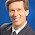## Tuesday, February 5, 2013

### Test of PDF Fit - Does not work

* I came up with this idea for testing if an observed distribution could be tested.

* The idea is that it standardized your data of interest and then tests if when the data is ordered if the difference between the empirical distribution of our data and your null distribution.

* The following program will sort the data and construct a pdf of our null (the normal in this case).
cap: program drop normal_check
program define normal_check
* Preverse the input data
preserve

* Sort from smallest to largest
sort x

* Standardize x
egen xnew = std(x)

* Create a CDF of what each obsrvations probability is under the null.
gen p = (_n-.5)/_N

* Create a pdf projection of what each bin's expected value is under the null.
gen targ_x = invnormal(p)

* Calculate the difference (which is what we will be testing.
gen dif_norm = xnew-targ_x

* Regress the difference on a constant
reg dif_norm

* If the option "graph" was specified then graph the hypathetical and the observed densities.
if "`1'" == "graph" twoway kdensity xnew || kdensity x

* Restore the data to how it was before initiating the program
restore
end

* Now let's generate some sample data
clear

set obs 100
gen x = rnormal()

normal_check graph
* We can see that our PDF fitness test has failed.

* Perhaps if we bootstrap the process?
bs: normal_check

* We reject the null (though we know it is true).

* Nope.  Why not?

* The sorting mechanism is a violation of the independence assumption.

* We need to generate alternative test statistics that will adjust for non-random sorting.

* My post tomorrow will demonstrate a response to this post which is generally effective at detecting underlying distributions.

* Distribution detection however is nothing new in statistics.

* Look up the ksmirnov command.  There is also Anderson-Darling and Chi-Squared options though I do not know what is coded in Stata or what their syntax looks like.

#### 1 comment:

1.I think what you are doing is related to the quantile-quantile plot, which enables you to compare the distibution of your data to a theoretical standardized distribution. For references and some SAS code that you can copy, see http://blogs.sas.com/content/iml/2011/10/28/modeling-the-distribution-of-data-create-a-qq-plot/